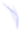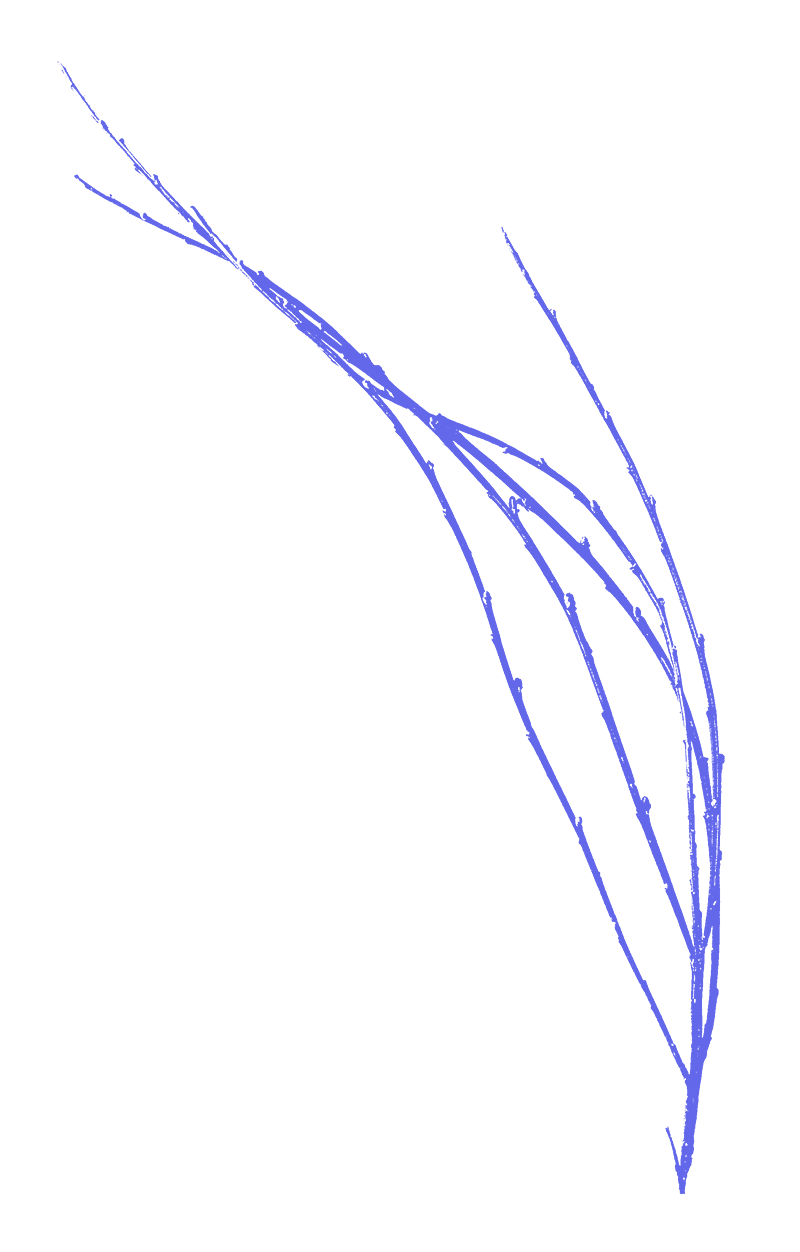## ((((((((((((((((((((((((((((((((((((((((((((((((((((((((((((((((((((((((((((((((((((((((((((((((((((((((((((((((((((((((((((((((((((((((((((((((((((((((((((((((((((((((((((((((((((((((((((((((((((((((Organise Here))))))))))))))))))))))))))))))))))))))))))))))))))))))))))))))))))))))))))))))))))))))))))))))))))))))))))))))))))))))))))))))))))))))))))))))))))))))))))))))))))))))))))))))))))))))))))))))))))))))))

BOARC was established to support the work of others. As it stands, we host many different types of research gatherings, workshops and self-organised study weeks. We love it when people push what’s possible with the space, and will always try and accommodate projects that sit in line with our values.

Please get in touch if you are looking for somewhere for your group to work so we can figure out scheduling together.Live-in Steward Role

Live-in stewards are invited to stay at BOARC for a period of 3 months to take advantage of the space to pursue their own practice without paying any nightly fees. In return, they take responsibility during the night for four or five nights per week. Positions begin in January, March, June and September on a rolling basis. If you are interested in this position, please send us an email and we’ll reply with the info pack and application procedure.

Co-steering BOARC as a project

BOARC is a young experimental project and we are responsively shaping the organisation as we go along. We really appreciate people getting to know the project and offering contributions.

Annual General Exchange

The AGM is held as part of the Annual General Exchange between 31st October - 5th November every year.

There are a couple of meetings in the year in which stayers and users of BOARC can offer thoughts and ideas for change. The team running BOARC will consider the ideas alongside the aims and values of the project and report back on any changes that have been implemented since the last meeting.

Heritage Education Centre Space (HECS)

We are currently developing a Heritage Education Centre Space (HECS). We periodically hold working group meetings to set up the HECS. More information on opportunities relating to this project will be posted here soon. To find out more please email: enquiries@bidstonobservatory.org.